# 前端快速生成 parent_id 关联的树结构数据

07/22 10:54

## 准备-测试数据生成代码

### 代码

``````// genTestData.js
function getRandomByRange(start, end) {
let length = end - start
return start + (Math.random() * length) >>> 0
}

function getRandomCode() {
return Math.random().toString(36).slice(2)
}

function getRandomCodeByData(data) {
return data[getRandomByRange(0, data.length)].code
}

export default function() {
let data = []
// 随机生成 20-30 个一级节点
for (let i = 0, l = getRandomByRange(20, 30); i < l; i++) {
data.push({
facode: '0',
code: getRandomCode()
})
}
// 随机生成 100-120 个随机关联节点
for (let i = 0, l = getRandomByRange(100, 120); i < l; i++) {
data.push({
facode: getRandomCodeByData(data),
code: getRandomCode()
})
}
return data.sort(() => (Math.random() - 0.5))
}
``````

### 测试

``````// demo.js
import genTestData from './genTestData.js'
const oriData = genTestData()
console.table(oriData)
``````## 常规生成方法

### 代码

``````// tree.js
export class TreeCreate {
constructor(treeNode, treeConfig) {
this.treeNode = treeNode
this.treeConfig = Object.assign({
fId: 'pid', // 关联的 parent_id 字段名
id: 'id', // 关联的 parent_id 的 id 字段名
rootId: '0', // 开始的 根节点关联的 parent_id 对应的 字段名
}, treeConfig)
this.treeData = [] // 树数据
}
// 树生成, 递归调用
createTree(data, parent = null) {
let config = this.treeConfig
let TreeNode = this.treeNode
let treeData, pid, i, l, val, children
treeData = []
for (i = 0, l = data.length; i < l; i++) {
val = data[i]
if (parent === null) {
pid = config.rootId
} else {
pid = parent[config.id]
}
if (val[config.fId] === pid) {
let t = {}
TreeNode.call(t, val)
children = this.createTree(data, Object.assign(t, val))
treeData.push(t)
treeData[treeData.length - 1].children = children
}
}
return treeData
}
// 生成树
create(data) {
this.treeData = this.createTree(data)
}
// 获取树状的树数据(深拷贝)
getTreeData() {
// 演示简易处理，无法处理循环引用对象，如果树节点需要保留父级引用，则需要改为可以处理循环引用的深拷贝方法
return JSON.parse(JSON.stringify(this.treeData))
}
}
``````

### 使用方式

`treeNode` 传入树节点数据生成函数，不能使用箭头函数，this为当前节点，默认空对象。 接受参数item为原始数据对象，根据需要为this对象赋值字段。如果简单需要全字段的，可以通过`Object.keys`遍历参数的所有字段，然后全部赋值到this的对应字段上。
`treeConfig` 为配置参数

• `FId` 关联的 parent_id 字段名
• `Id` 关联的 parent_id 的 id 字段名
• `rootId` 开始的 根节点关联的 parent_id 对应的 字段名

### 测试性能

``````import genTestData from './genTestData.js'
import { TreeCreate } from './tree.js'
// 测试数据
const oriData = genTestData()
console.log(`树节点数量 \${oriData.length}`)

// 树
let demoTree = new TreeCreate(function(item) {
for (let key of Object.keys(item)) {
this[key] = item[key]
}
}, { fId: 'facode', id: 'code', rootId: '0' })

console.time('生成树时间')
demoTree.create(oriData, true)
console.timeEnd('生成树时间')
``````

#### 百级数据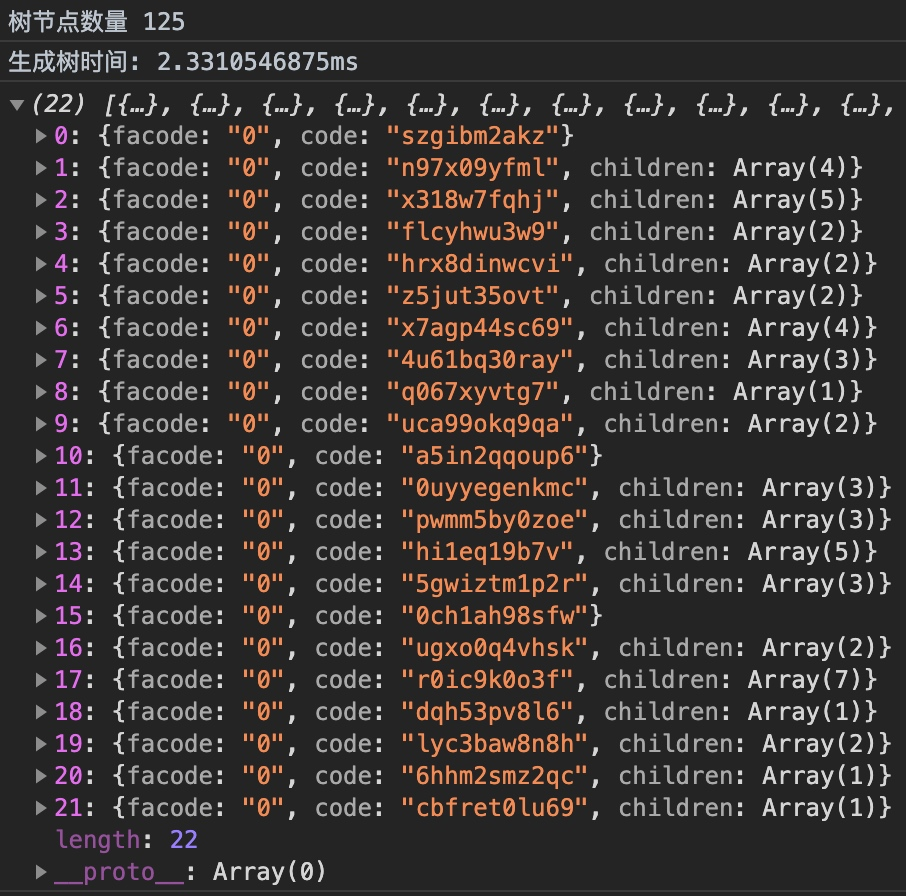#### 千级数据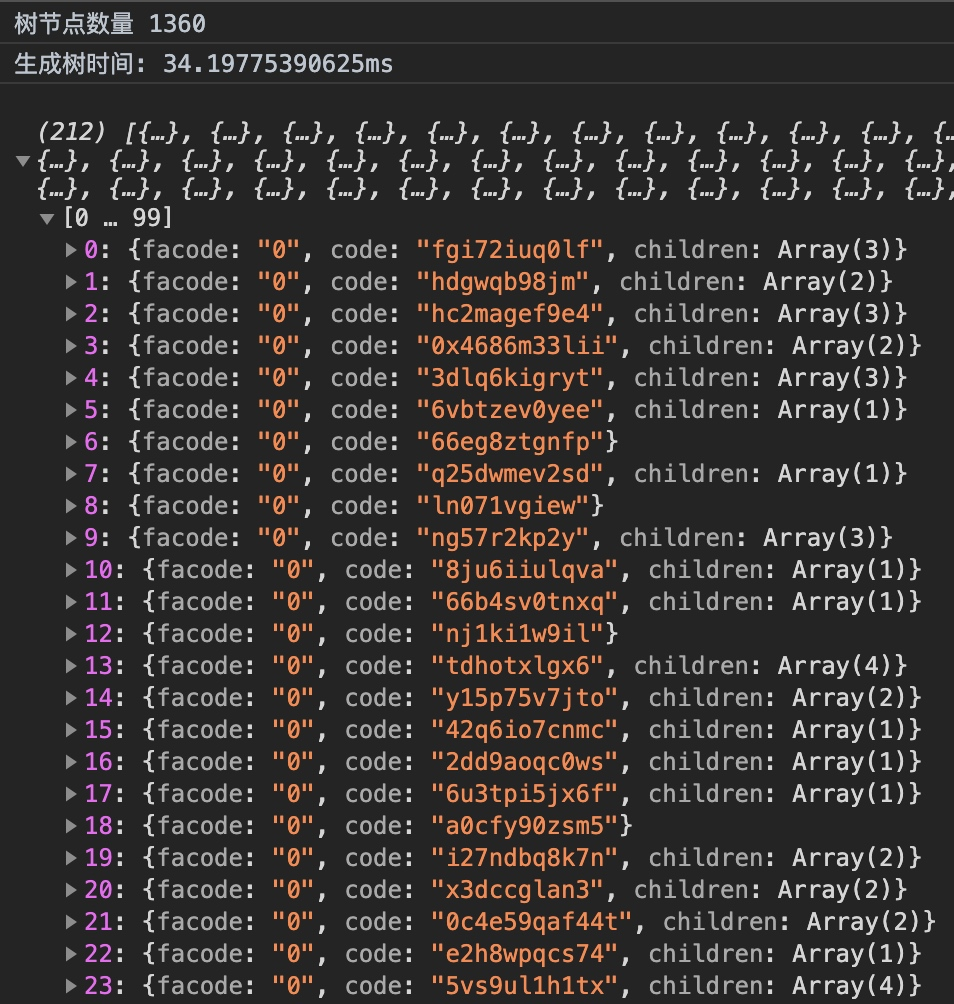#### 万级数据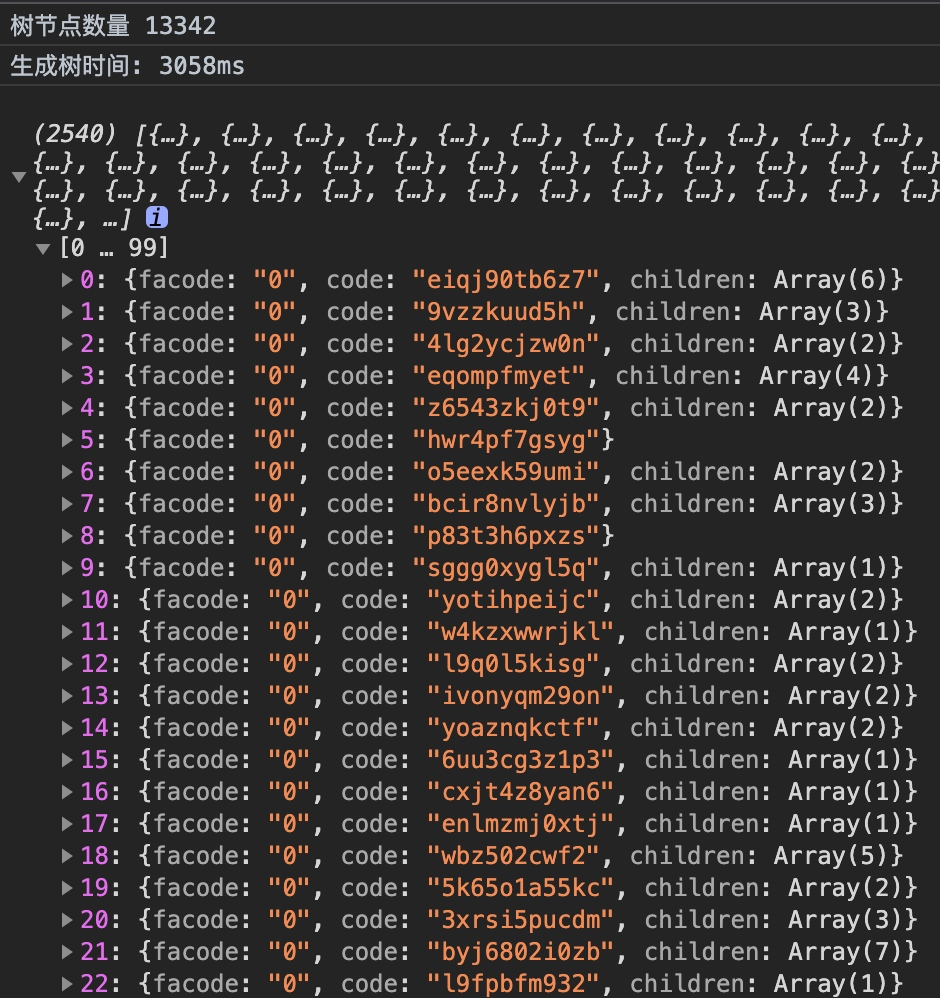## 快速生成方法

### 代码

``````// tree.js
export class TreeCreateFast {
constructor(treeNode, treeConfig) {
this.treeNode = treeNode
this.treeConfig = Object.assign({
fId: 'pid', // 关联的 parent_id 字段名
id: 'id', // 关联的 parent_id 的 id 字段名
rootId: '0', // 开始的 根节点关联的 parent_id 对应的 字段名
}, treeConfig)
this.treeData = [] // 树状的树数据
}
// 按 fId 分组
groupBy(data) {
let config = this.treeConfig
let group = []
data.forEach(v => {
let key = v[config.fId]
if (!group.hasOwnProperty(key)) {
group[key] = [v]
} else {
group[key].push(v)
}
})
return group
}
// 树生成, 递归调用
createTree(data, parent = null) {
let config = this.treeConfig
let TreeNode = this.treeNode
let treeData, pid, children
treeData = []
if (parent === null) {
pid = config.rootId
} else {
pid = parent[config.id]
}
if (data.hasOwnProperty(pid)) {
data[pid].forEach(val => {
let t = {}
TreeNode.call(t, val)
children = this.createTree(data, Object.assign(t, val))
treeData.push(t)
treeData[treeData.length - 1].children = children
})
}
return treeData
}
// 生成树
create(data) {
this.treeData = this.createTree(this.groupBy(data))
}
// 获取树状的树数据(深拷贝)
getTreeData() {
// 演示简易处理，无法处理循环引用对象，如果树节点需要保留父级引用，则需要改为可以处理循环引用的深拷贝方法
return JSON.parse(JSON.stringify(this.treeData))
}
}
``````

### 测试性能

``````import genTestData from './genTestData.js'
import { TreeCreateFast } from './tree.js'
// 测试数据
const oriData = genTestData()
console.log(`树节点数量 \${oriData.length}`)

// 树
let demoTree = new TreeCreateFast(function(item) {
for (let key of Object.keys(item)) {
this[key] = item[key]
}
}, { fId: 'facode', id: 'code', rootId: '0' })

console.time('生成树时间')
demoTree.create(oriData, true)
console.timeEnd('生成树时间')
``````

#### 万级数据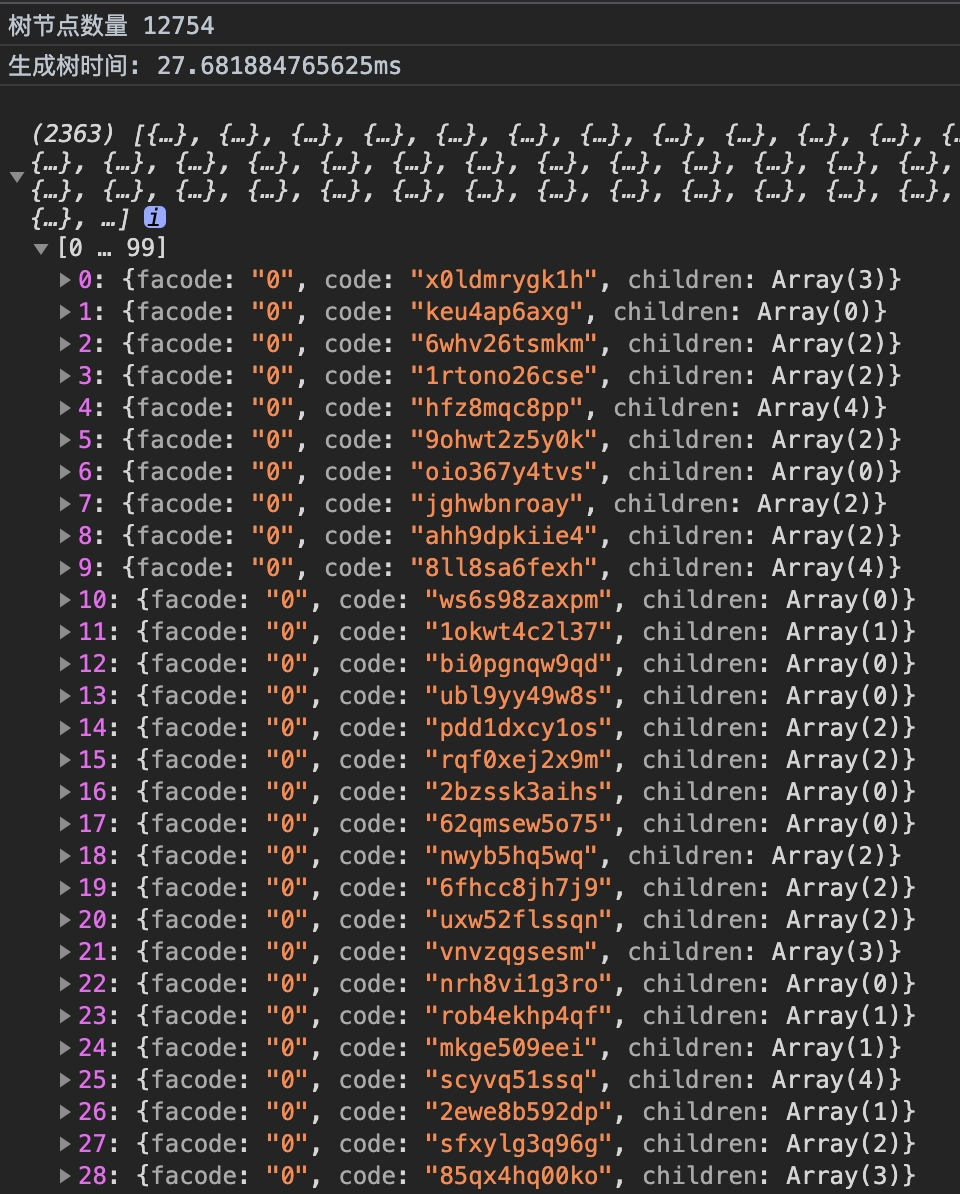#### 十万级数据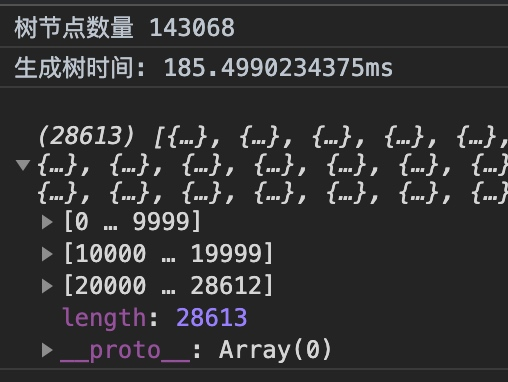#### 挑战下百万级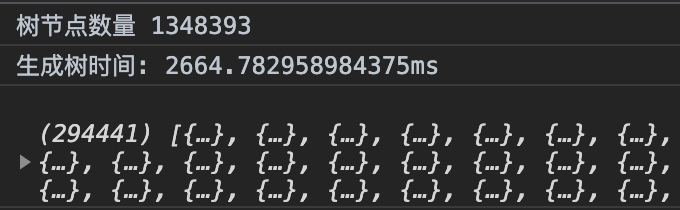## 总结

0
0 收藏

### 作者的其它热门文章0 评论
0 收藏
0